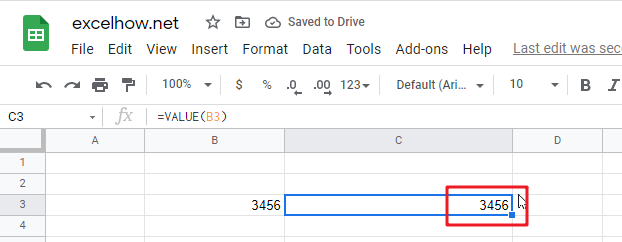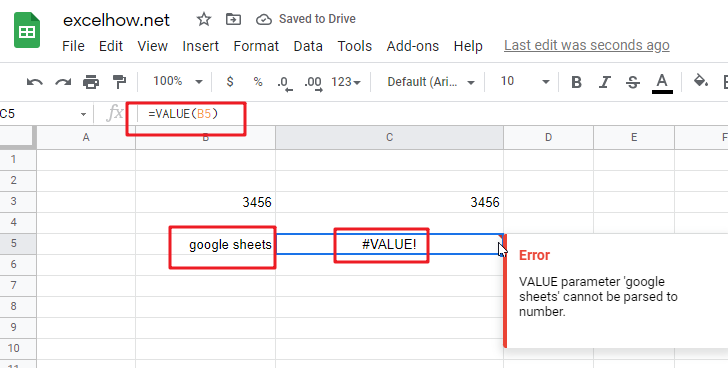# ExcelHow

This post will guide you how to use Google Sheets VALUE function with syntax and examples.

## Description

The Google Sheets VALUE function converts a text string that represents a number to a numeric value.

The VALUE function can be used to converts a text string in the date, time or number formats into a numeric value in google sheet. The purpose of this function is to convert text to a number and its returned values is a numeric value.

The VALUE function is a build-in function in Google Sheets and it is categorized as a Text Function.

## Syntax

The syntax of the VALUE function is as below:

= VALUE (text)

Where the VALUE function argument is:

• Text -This is a required argument. The text string that you want to convert it to a number.

Note:

• Text can be in any of the constant number, date, or time formats. And if text is not a number, the VALUE function will return #VALUE! Error.
• If the VALUE function is not able to convert a text value to a numeric result, it returns a #VALUE! Error message.
• VALUE function is provided for compatibility with other spreadsheet applications.

## Google Sheets VALUE Function Examples

The below examples will show you how to use google sheets VALUE function to convert string to a number.

#1 To convert a text in B3 cell to a number, just using formula:

`= VALUE(B3)`#2 to convert a text string that is not a recognized format (number, date, time format) into a numeric value, using the below formula:

`=VALUE(B5)`You will see that the #VALUE! Error will be returned by the VALUE function. Because the text is not a number.